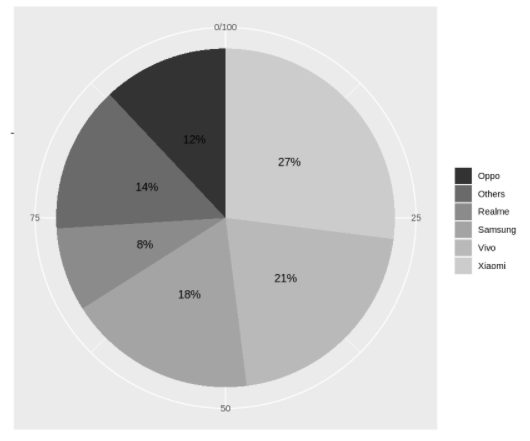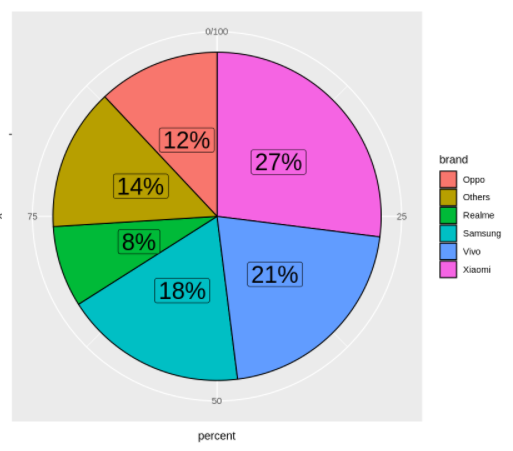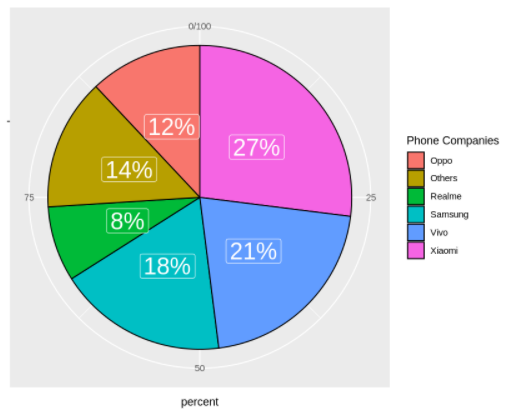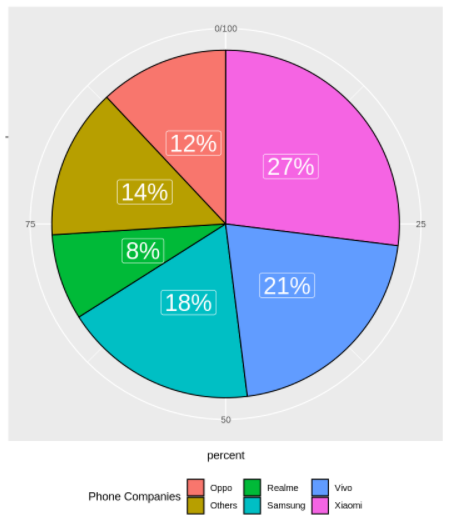# Tutorial for Pie Chart in ggplot2 with Examples### Dataset

We are going to create our own toy sample dataset of mobile phone companies and their market share. This is loaded into a data frame ‘data’ that will be used throughout the ggplot2 pie chart examples.

Out:

### Example 4: Applying Gray Scale to Pie Chart using scale_fill_grey()

If we want to opt for a grey color palette for coloring pies of the pie chart in ggplot2, then we can use scale_fill_grey() as shown in the below example.

In :

```ggplot(data, aes(x="", y=percent, fill=brand)) +
geom_bar(stat="identity", width=1) +
coord_polar("y", start=0) +
geom_text(aes(label = paste0(percent, "%")), position = position_stack(vjust=0.5)) +
labs(x =NULL, y = NULL, fill = NULL) +
scale_fill_grey()```

Out:Out:
Out:Out:

The legend title can be customized with guides() layer as shown in the below example where the title has been changed to “Phone Companies”

In :

```ggplot(data, aes(x = "",y=percent, fill=brand)) +
geom_col(color = "black") +
geom_label(aes(label = paste0(percent, "%")), color = "white", position = position_stack(vjust = 0.5), size=8, show.legend = FALSE) +
coord_polar(theta = "y") +
guides(fill = guide_legend(title = "Phone Companies"))```

Out:The legend can be placed at several places, possible placement values are “bottom”, “left”, “top” and “right” (default). To place the legend at the bottom use the legend.position = “bottom” in the theme layer.

Out:Out: